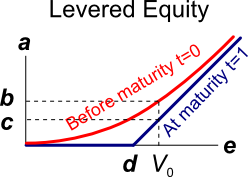# Fight Finance

#### CoursesTagsRandomAllRecentScores

A European bond paying annual coupons of 6% offers a yield of 10% pa.

Convert the yield into an effective monthly rate, an effective annual rate and an effective daily rate. Assume that there are 365 days in a year.

All answers are given in the same order:

$$r_\text{eff, monthly} , r_\text{eff, yearly} , r_\text{eff, daily}$$

Which statement is the most correct?

Three important classes of investable risky assets are:

• Corporate debt which has low total risk,
• Real estate which has medium total risk,
• Equity which has high total risk.

Assume that the correlation between total returns on:

• Corporate debt and real estate is 0.1,
• Corporate debt and equity is 0.1,
• Real estate and equity is 0.5.

You are considering investing all of your wealth in one or more of these asset classes. Which portfolio will give the lowest total risk? You are restricted from shorting any of these assets. Disregard returns and the risk-return trade-off, pretend that you are only concerned with minimising risk.

A risky firm will last for one period only (t=0 to 1), then it will be liquidated. So it's assets will be sold and the debt holders and equity holders will be paid out in that order. The firm has the following quantities:

$V$ = Market value of assets.

$E$ = Market value of (levered) equity.

$D$ = Market value of zero coupon bonds.

$F_1$ = Total face value of zero coupon bonds which is promised to be paid in one year.The levered equity graph above contains bold labels a to e. Which of the following statements about those labels is NOT correct?

According to option theory, it's rational for students to submit their assignments as or as possible?

A 'fully amortising' loan can also be called a:

An investor wants to make a portfolio of two stocks A and B with a target expected portfolio return of 6% pa.

• Stock A has an expected return of 5% pa.
• Stock B has an expected return of 10% pa.

What portfolio weights should the investor have in stocks A and B respectively?

A 2-year futures contract on a stock paying a continuous dividend yield of 3% pa was bought when the underlying stock price was $10 and the risk free rate was 10% per annum with continuous compounding. Assume that investors are risk-neutral, so the stock's total required return is the risk free rate. Find the forward price $(F_2)$ and value of the contract $(V_0)$ initially. Also find the value of the contract in 6 months $(V_{0.5})$ if the stock price rose to$12.

Which of the following statements about an option (either a call or put) and its underlying stock is NOT correct?

 European Call Option on a non-dividend paying stock Description Symbol Quantity Spot price ($) $S_0$ 20 Strike price ($) $K_T$ 18 Risk free cont. comp. rate (pa) $r$ 0.05 Standard deviation of the stock's cont. comp. returns (pa) $\sigma$ 0.3 Option maturity (years) $T$ 1 Call option price ($) $c_0$ 3.939488 Delta $\Delta = N[d_1]$ 0.747891 $N[d_2]$ $N[d_2]$ 0.643514 Gamma $\Gamma$ 0.053199 Theta ($/year) $\Theta = \partial c / \partial T$ 1.566433

Question 984  principal agent problem, moral hazard, asymmetric information, no explanation

When does the ‘principal-agent problem’ occur? Is it when:

I. The principal has conflicting incentives (moral hazard);

II. The agent has conflicting incentives (moral hazard);

III. The principal has incomplete information about the agent (asymmetric information); or

IV. The agent has incomplete information about the principal (asymmetric information)?

The principal-agent problem occurs when statements: